Question

# A straight, nonconducting plastic wire 8.50 cm long carries a charge density of 130 nC/m distributed...

A straight, nonconducting plastic wire 8.50 cm long carries a charge density of 130 nC/m distributed uniformly along its length. It is lying on a horizontal tabletop.

Part a) Find the magnitude and direction of the electric field this wire produces at a point 5.00 cm directly above its midpoint.

Part b) Is electric field directed upward or downward

Part c) If the wire is now bent into a circle lying flat on the table, find the magnitude and direction of the electric field it produces at a point 5.00 cm directly above its center.

Part d) Is electric field directed upward or downward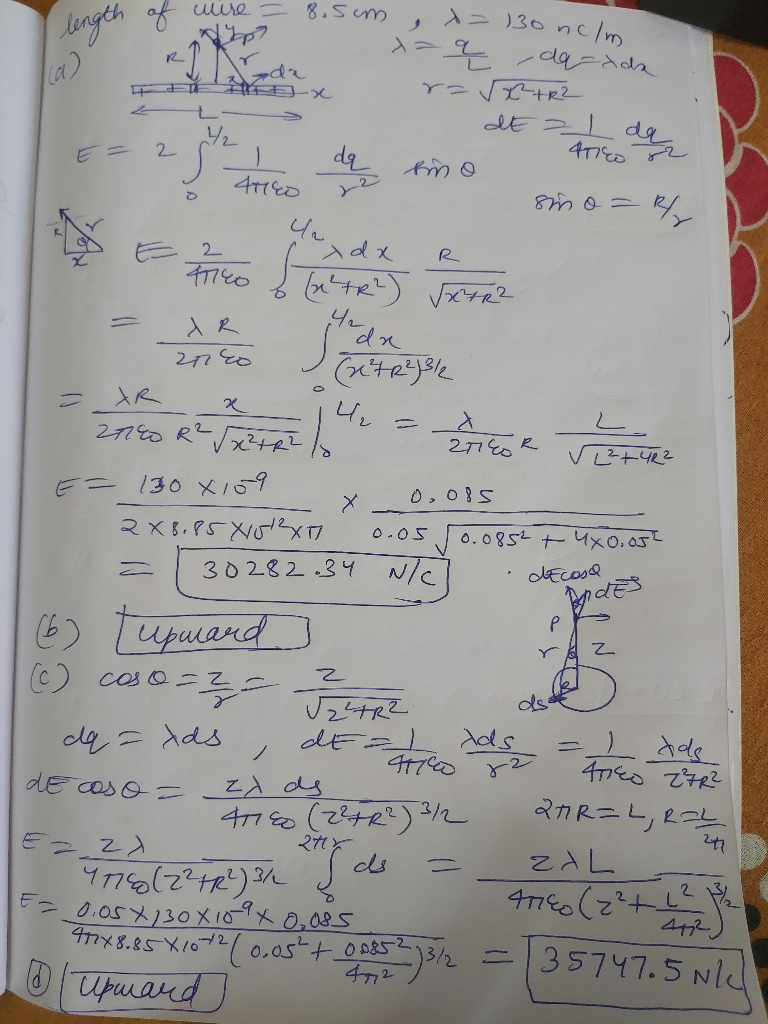#### Earn Coins

Coins can be redeemed for fabulous gifts.

Similar Homework Help Questions
• ### A straight, nonconducting plastic wire 8.50 cm long carries a charge density of +175 nC/m distributed uniformly along its length.

A straight, nonconducting plastic wire 8.50 cm long carries a charge density of +175 nC/m distributed uniformly along its length. It is lying on a horizontal tabletop. (a) Find the magnitude and direction of the electric field this wire produces at a point 6.00 cm directly above its midpoint (b) If the wire is now bent into a circle lying flat on the table, find the magnitude and direction of the electric field it produces at a point 6.00 cm...

• ### A straight, nonconducting plastic wire 9.00 cm long carries a charge density of 100 nC/m distributed...

A straight, nonconducting plastic wire 9.00 cm long carries a charge density of 100 nC/m distributed uniformly along its length. It is lying on a horizontal tabletop.​ A) Find the magnitude and direction of the electric field this wire produces at a point 4.00 cm directly above its midpoint.​ B) If the wire is now bent into a circle lying flat on the table, find the magnitude and direction of the electric field it produces at a point 4.00 cm...

• ### A straight, nonconducting plastic wire 8.50 cm long carries a charge density of 100 nC/m distributed uniformly along its length. It is lying on a horizontal tabletop.

A straight, nonconducting plastic wire 8.50 cm long carries a charge density of 100 nC/m distributed uniformly along its length. It is lying on a horizontal tabletop.  Part C  If the wire is now bent into a circle lying flat on the table, find the magnitude and direction of the electric field it produces at a point 4.50 cm directly above its center.

• ### Constants Part A A straight, nonconducting plastic wire 7.00 cm long carries a charge density of...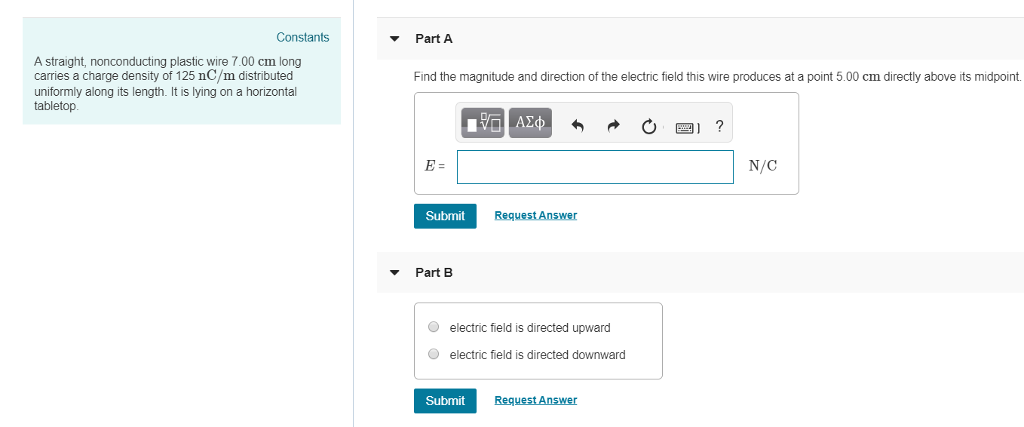Constants Part A A straight, nonconducting plastic wire 7.00 cm long carries a charge density of 125 nC/m distributed uniformly along its length. It is lying on a horizontal tabletop. Find the magnitude and direction of the electric field this wire produces at a point 5.00 cm directly above its midpoint. E: N/C Submit Request Answer Part B O electric field is directed upward O electric field is directed downward Submit Request Answer We were unable to transcribe this image

• ### A straight, nonconducting plastic wire.

A straight, nonconducting plastic wire 8.50 long carries a charge density of 130 distributed uniformly along its length. It is lying on a horizontaltabletop.a) Find the magnitude and direction of the electric field this wire produces at a point 6.00 directly above its midpoint.b) If the wire is now bent into a circle lying flat on the table, find the magnitude and direction of the electric field it produces at a point 6.00 directly aboveits center.

• ### A straight, nonconducting plastic wire 9.00 long carries a charge density of 125 distributed uniformly along...

A straight, nonconducting plastic wire 9.00 long carries a charge density of 125 distributed uniformly along its length. It is lying on a horizontal tabletop.

• ### Figure shows a thin nonconducting wire, carrying a total charge of Q = 15.0 nC, uniformly...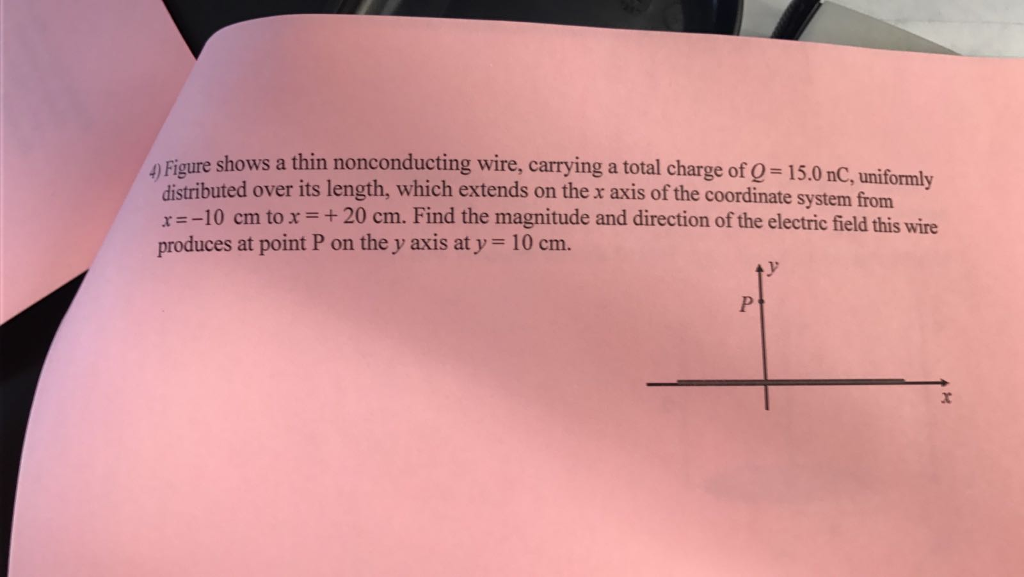Figure shows a thin nonconducting wire, carrying a total charge of Q = 15.0 nC, uniformly distributed over its length, which extends on the x axis of the coordinate system from x = -10 cm to x = + 20 cm. Find the magnitude and direction of the electric field this wire produces at point P on the y axis at y = 10 cm.

• ### A nonconducting solid sphere of radius 2.10 cm carries a uniformly distributed positive charge of 8.50...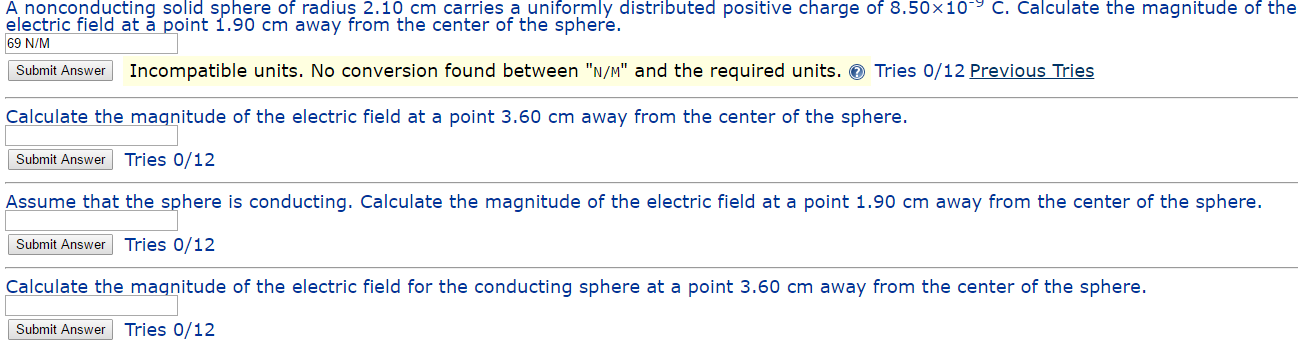A nonconducting solid sphere of radius 2.10 cm carries a uniformly distributed positive charge of 8.50 times 10^-9 C. Calculate the magnitude of the electric field at a point 1.90 cm away from the center of the sphere. Incompatible units. No conversion found between "N/M" and the required units. Calculate the magnitude of the electric field at a point 3.60 cm away from the center of the sphere. Assume that the sphere is conducting. Calculate the magnitude of the electric...

• ### Part A Two very large, nonconducting plastic sheets, each 10.0 cm thick, carry uniform charge densities...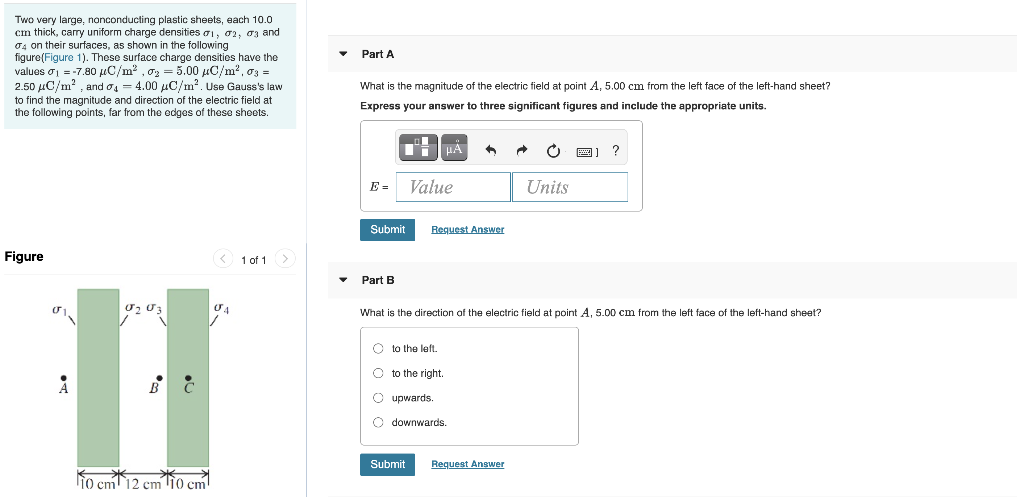Part A Two very large, nonconducting plastic sheets, each 10.0 cm thick, carry uniform charge densities 01, 02, 03 and 04 on their surfaces, as shown in the following figure(Figure 1). These surface charge densities have the values 01 = -7.80 C/m², 03 = 5.00 4C/m², 03 = 2.50 4C/m², and 04 = 4.00 4C/m². Use Gauss's law to find the magnitude and direction of the electric field at the following points, far from the edges of these sheets. What...

• ### A particle has a charge of -3.10 nC . A.)Find the magnitude of the electric field...

A particle has a charge of -3.10 nC . A.)Find the magnitude of the electric field due to this particle at a point 0.235 m directly above it. B.) Find the direction of the electric field at this point. (upward or downward) C.) At what distance from the particle does its electric field have a magnitude of 10.5 N/C ?